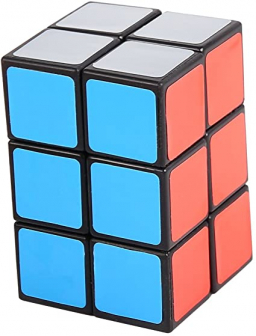# Capacity 70404

The box is 55 cm high and 40 cm wide. The capacity of the box is 180 liters. How much does the box length measure? And what is the capacity of the box in cm cubic?

a =  81.8182 cm
V =  180000 cm3

### Step-by-step explanation:Did you find an error or inaccuracy? Feel free to write us. Thank you!

Tips for related online calculators
Need help calculating sum, simplifying, or multiplying fractions? Try our fraction calculator.
Do you know the volume and unit volume, and want to convert volume units?